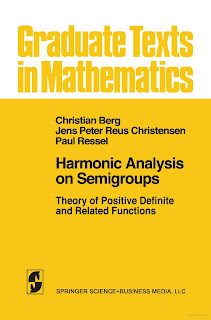APPLIED MATHEMATICS

# Harmonic Analysis on Semigroups Theory of Positive Definite and Related Functions Free DownloadThe Fourier transform and the Laplace transform of a positive measure share, together with its moment sequence, a positive definiteness property which under certain regularity assumptions is characteristic for such expressions. This is formulated in exact terms in the famous theorems of Bochner, Bernstein-Widder and Hamburger. All three theorems can be viewed as special cases of a general theorem about functions qJ on abelian semigroups with involution (S, +, *) which are positive definite in the sense that the matrix (qJ(sJ + Sk» is positive definite for all finite choices of elements St, . . . , Sn from S. The three basic results mentioned above correspond to (~, +, x* = -x), ([0, 00[, +, x* = x) and (No, +, n* = n). The purpose of this book is to provide a treatment of these positive definite functions on abelian semigroups with involution. In doing so we also discuss related topics such as negative definite functions, completely mono tone functions and Hoeffding-type inequalities. We view these subjects as important ingredients of harmonic analysis on semigroups. It has been our aim, simultaneously, to write a book which can serve as a textbook for an advanced graduate course, because we feel that the notion of positive definiteness is an important and basic notion which occurs in mathematics as often as the notion of a Hilbert space.